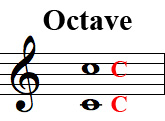# octave

###### AHK-tiv

[English]

An interval spanning seven diatonic degrees, eleven semitones. An octave above C would be C. The frequency of a note one octave above another will have exactly twice as many Hertz as the frequency of the note an octave below it. In the example below, Middle C (the lower note) is 261.63 hertz and the C shown an octave higher is 523.25 hertz.

See more about pitch frequencies in the Appendix.

### Example